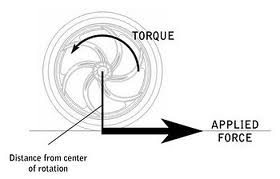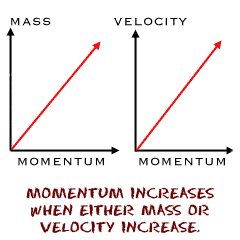# Difference between Momentum and Torque

##### Key Difference: Torque (moment or moment of force), the word has been derived from Latin which means ‘to twist’. It is a tendency which measures the amount of force which acts upon a body in order to rotate it about a pivot or axis. Momentum is a property possessed by moving objects and is directly proportional to both mass and speed of the body. It can be described as a quantity which is associated with an object’s resistance to stopping.Torque (moment or moment of force), the word has been derived from Latin which means ‘to twist’. It is a tendency which measures the amount of force which acts upon a body in order to rotate it about a pivot or axis. It is a vector quantity. If somebody pushes a door to open it,  the door rotates about its hinges. In this example, torque is being used. It can be referred to as the turning effectiveness of a force. The formula used for the calculation of torque is

T = r XF = rFsin(a)

(It is a cross product between the distance vector (distance from pivot point to the point where the force is being applied) and the force vector) and a is the angle between r and F.

The direction of Torque is determined by using right hand rule – direct fingers towards r and then curl towards the direction of F. In this particular instance, the thumb will be pointing towards the direction of Torque. The SI unit of torque is a Newton-meter.Momentum is another vector quantity. It is a property possessed by moving objects and is directly proportional to both mass and speed of the body. An object with a greater momentum is hard to stop than in comparison to an object with a lesser momentum. Thus, it can be described as a quantity which is associated with an object’s resistance to stopping. One can also refer to it as a type of moving inertia. The direction of momentum is the same as the velocity of the object.

Momentum =p=mv (mass * velocity)

The SI unit of momentum is kilogram meter per second. [kg m/s]

Torque can also be defined in terms of angular momentum as the rate of change of angular momentum.

Comparison between Momentum and Torque:

 Momentum Torque Definition It is a property possessed by moving objects and is directly proportional to both mass and speed of the body. It is a tendency which measures the amount of force which acts upon a body in order to rotate it about a pivot or axis. It is a vector quantity. Formula Momentum =p=mv (mass * velocity) T = r XF = rFsin(a)  (It is a cross product between the distance vector (distance from pivot point to the point where the force is being applied) and the force vector) and a is the angle between r and F. Direction Same as the velocity of the object. It is determined by using right hand rule Unit The SI unit of momentum is kilogram meter per second. [kg m/s] Newton-meter Properties It is conserved when there are no external forces acting.  It helps in understanding collisions. It is calculated with respect to (about) a point. A change in the point changes the magnitude and direction of the torque.

Image Courtesy: physics4kids.com, vexrobotics.com